Two friends

Peter can do all his work himself in 6 hours. Martin can do the same work himself in 8 hours. Peter worked first and then replaced by Martin. Whole work was done in 6.5 hours. Calculate how long Peter worked before replaced by Martin.

Result

p =  4.5 h

Solution:

p/6 + (6.5-p)/8 = 1

p = 4.5

p = 92 = 4.5

Calculated by our simple equation calculator.

Leave us a comment of this math problem and its solution (i.e. if it is still somewhat unclear...):Be the first to comment!To solve this verbal math problem are needed these knowledge from mathematics:

Need help calculate sum, simplify or multiply fractions? Try our fraction calculator. Do you have a linear equation or system of equations and looking for its solution? Or do you have quadratic equation?

Next similar math problems:

1. NovakNovak needed to dig up three of the same pit in the garden. The first pit dug father alone for 15 hours. His second dig son helped him and it did that in six hours. The third pit dug son himself. How long it took him?
2. Painters 4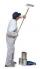One painter will do the job for 12 days. After a 4 1/3 day he comes second to help him and complete work together for 8 days. How long would work the second painter himself?
3. Three peopleThree people start doing a work at a same time. The first worked only 2 hours. The second ended 3 hours before the end. On an individual basis, it would take the first time to do the work 10 hours, second 12 hours and 15 hours third. How many hours did it.
4. Trucks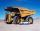Truck take you the some amount of clay in 30 hours. Second truck in 40 hours. Trucks carted the clay away some time together then the second truck broke down and the first one completed the job in five hours. What time worked together?
5. Hectoliters of waterThe pool has a total of 126 hectoliters of water. The first pump draws 2.1 liters of water per second. A second pump pumps 3.5 liters of water per second. How long will it take both pumps to drain four-fifths of the water at the same time?
6. Six workersSix workers planned to repair the roof in 5 days. After two days, two workers get sick. How long will the remaining workers complete the roof at the same rate of work?
7. Tonic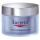One package tonic consume Mom 12 weeks and daughter for 14 weeks. 4 weeks they use the same cream together and then only daughter. How many weeks endured tonic to daughter then?
8. Two typists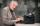There are two typists who are rewriting the material 814 pages. First can it handle rewrite yourself for 24 days; the second 12 days. First typist wrote material yourself 4 days rest rewrites yourself second typist. How many days will it take rewriting alt
9. Digging companies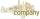Company A would dig a pit for 12 days, company B for 15 days, company C for 20 days and the company D for 24 days. Work began together companies C and D, but after three days joined them other two companies. How long took to dig a pit?
10. Our houseOur house would paint four painters in 14 days. How long could it paint five painters? How long will it take if two other painters will come to help after 5 days?
11. The tank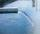The tank had 9 inflows to be filled in 21 days. After 9 days, 3 trips out. How many days did the remaining 6 tributaries fill the tank?
12. Find the 7Find the number that is smaller than 5 5/12 by as much as 2 2/13 is smaller than 6 1/6
13. Holidays - on poolChildren's tickets to the swimming pool stands x € for an adult is € 2 more expensive. There was m children in the swimming pool and adults three times less. How many euros make treasurer for pool entry?
14. Six yearsIn six years Jan will be twice as old as he was six years ago. How old is he?
15. Simple equation 6Solve equation with one variable: X/2+X/3+X/4=X+4
16. Fifth of the numberThe fifth of the number is by 24 less than that number. What is the number?
17. Equation with mixed fractions2 3/5 of 1430+? = 1900. How to do this problem